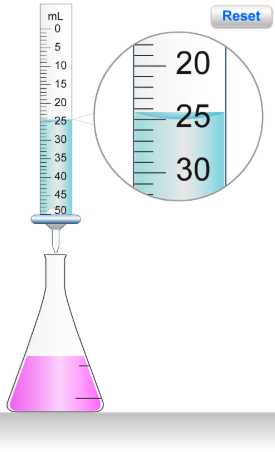# Problem: The flask shown here contains 10.0 mL of HCl and a few drops of phenolphthalein indicator. The buret contains 0.310 M NaOH.What volume of NaOH is needed to reach the end point of the titration? What was the initial concentration of HCI?

###### FREE Expert Solution

In this problem, we are asked to determine the (a) volume of NaOH is needed to reach the endpoint of the titration and the (b) initial concentration of HCI.

Part A. From the image:

Lower meniscus in the buret → 25.0 mL

Thus, the volume of NaOH used is 25.0 mL NaOH.

89% (364 ratings)###### Problem Details

The flask shown here contains 10.0 mL of HCl and a few drops of phenolphthalein indicator. The buret contains 0.310 M NaOH.What volume of NaOH is needed to reach the end point of the titration?

What was the initial concentration of HCI?